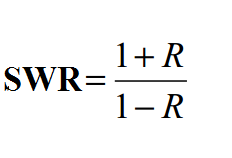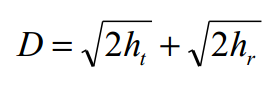# Chapter 9: Antennas and Transmission Lines – Review Notes

(Last Updated On: December 8, 2017)This is the summary notes of the important terms and concepts in Chapter 9 of the book COMMUNICATIONS ELECTRONICS by Louis E. Frenzel. This book introduces basic communication concepts and circuits, including modulation techniques, radio transmitters and receivers. It also discusses antennas and microwave techniques at a technician level and covers data communication techniques (modems, local area networks, fiber optics, satellite communication) and advanced applications (cellular telephones, facsimile and radar). The work is suitable for courses in Communications Technology. The notes are properly synchronized and concise for much better understanding of the book. Make sure to familiarize this review notes to increase the chance of passing the ECE Board Exam.

 CHAPTER 9 Antennas and Transmission Lines

1. A transmission line is a two-wire cable used to carry RF energy between two different pieces of communications equipment or between an antenna and a receiver or transmitter.

2. The two most common types of transmission lines are balanced and coaxial.

3. The primary feature of a transmission line is its characteristic or surge impedance Zo which is a function of the distributed inductance L and the capacitance C per unit length (Zo = L / C).

4. The characteristic impedance of a balanced line is determined by its physical dimensions. [ Zo =276 log (2 S I D) where S is the center-to-center spacing of the conductors and D is the diameter of the conductors.]

5. The characteristic impedance of coax also depends on its physical dimensions. [ Zo = 138 log (D I d) where D is the inside diameter of the shield and d is the diameter of the inner conductor.]

6. The proper use of a transmission line is to terminate it in a load impedance equal to its surge impedance. All the power applied to the line will be absorbed by the load.

7. Wavelength is the distance between adjacent peaks of a RF wave. It is also the distance traveled by a signal in one cycle. Wavelength (λ) is computed with the expression λ = 300 / f where f is the frequency in megahertz and is in meters, Or λ = 984 / f where A is in feet.

8. If a transmission line is not terminated in its characteristic impedance, the load will not absorb all the power. Some of it will be reflected back toward the generator.

9. If the load on a transmission line is an open or short, all the power applied to the line will be reflected back to the generator.

10. The forward or incident power applied to the line combines with the reflected power to produce a pattern of voltage and current variations along the line known as standing waves.

11. If the load impedance matches the line impedance, there are no standing waves.

12. A measure of the mismatch between line and load impedances or the maximum and minimum voltage and current variations along, the line is the standing-wave ratio (SWR) which is a number always greater than 1.

13. The SWR indicates how much power is delivered to the load and lost in the line. With SWR = 1, all power is delivered to the load.

14. The ratio of the reflected voltage V, to the incident voltage V, on a transmission line is caIled the reflection coefficient R (R = Vr I Vi). A properly terminated line will have R = O. A shorted or open line will have R =1.

15. The SWR in terms of the reflection coefficient is16. The SWR = ZO I ZL, or ZL l Zo where ZO is the characteristic impedance and ZL is the load impedance.

17. Every effort is made to reduce the SWR by using impedance-matching circuits to ensure that maximum power is delivered to the load.

18. Transmission lines, one-quarter or one-half wavelength long and either shorted or open, act like resonant or reactive circuits.

19. At UHF and microwave frequencies where one-half wavelength this less than 1 ft., transmission lines are commonly used to replace conventional LC tuned circuits.

20. A shorted quarter wave and an open half wave act like a parallel resonant circuit.

21. Both an open quarter-wave circuit and a shorted half-wave circuit act like a series resonant circuit.

22. The velocity of propagation of a radio signal is slower in a transmission line than in free space. This difference is expressed as the velocity factor F for different types of lines. Coax has a velocity factor of 0.6 to 0.7. The velocity factor of open wire line or twin lead is in the 0.7 to 0.8 range. In computing the length of a transmission line at a specific frequency, the velocity factor must be considered ( = 984 F/f).

23. An antenna or aerial is one or more conductors used to transmit or receive radio signals.

24. A radio signal is electromagnetic energy made up of electric and magnetic fields at right angles to one another and to the direction of signal propagation.

25. The polarization of a radio signal is defined as the orientation of the electric field with respect to the earth and is either vertical or horizontal.

26. The most common antenna is the half wave dipole or doublet that has a characteristic impedance of approximately 73 flat the center. Its length in feet is 468/f where f is the frequency in megahertz.

27. The dipole has a bidirectional figure-eight radiation pattern and is usually mounted horizontally but may also be used vertically.

28. A popular variation of the dipole is the folded dipole which is one-half wavelength long and has an impedance of 300 .

29. Another popular antenna is the quarter-wave vertical. The earth acts as the other quarter wave to simulate a half-wave vertical dipole.

30. The quarter-wave vertical is referred to as a ground plane antenna. It is fed with coax with the center conductor connected to the antenna and the shield connected to earth ground, to an array of quarter-wave, wires m called radials, or to a large, flat, metal surface. Its length in feet is 234/f where f is in megahertz.

31. The characteristic impedance of a ground plane is about 36.5. It has an omni directional radiation pattern that sends or receives equally well in all directions.

32. A directional antenna is one that transmits or receives over a narrow range in only one direction.

33. Directional antennas made up of two or more elements focus the radiation into a narrow beam, thus giving the antenna gain.

34. The gain of the, antenna is the power amplification resulting from the concentration of power in one direction. The gain may be expressed as a power ratio or in decibels.

35. The effective radiated power (ERP) of an antenna is the power input multiplied by the antenna power gain.

36. Directional antennas with two or more elements are caIled arrays. There are two types of arrays: parasitic and driven.

37. Parasitic elements called reflectors and directors when spaced parallel to a half wave dipole driven element help focus the signal into a narrow beam.

38. The measure of the directivity of an antenna is the beam width or beam angle measured in degrees.

39. A parasitic array made up of a driven element, reflector, and one or more directors is known as a Yagi or beam antenna and has a gain of 10 to 20 dB with a beam width of 40° to 20°.

40. Driven arrays consist of two or more half wavelength elements, each receiving power from the transmission line.

41. The three most popular driven arrays are the collinear, end-fire, and broadside.

42. A widely used driven array is the log periodic antenna which exhibits gain, directivity, and a wide operating frequency range.

43. A radio wave propagates through space in one of three ways: ground wave, sky waves, or direct waves.

44. The ground or surface wave leaves the antenna and follows the curvature of the earth. The ground wave is only effective on frequencies below 3 MHz.

45. The skywave propagates from the antenna upward where it is bent back to earth by the ionosphere.

46. The ionosphere is a portion of the earth’s atmosphere 30 to 250 mi above the earth that has been ionized by the sun.

47. The ionosphere is made up of three layers of different ionization density: the D, E, and F layers. The F layer is the most highly ionized and causes refraction or bending of radio waves back to earth.

48. The refraction of the ionosphere causes a radio signal to be bent back to earth with little or no attenuation long distances from the transmitter. This is known as a skip or hop.

49. Multiple skips or hops between the ionosphere and earth permit very long distances, even worldwide, communications. This effect is useful over the 3 – to 30-MHz range.

50. At frequencies above 30 MHz, propagation is primarily by the direct or space wave which travels in a straight line between transmitting and receiving antennas. This is known as line-of-sight communications.

51. Radio waves ate easily blocked or reflected by large objects. This is particularly true of VHF, UHF, and microwave signals.

52. The communications distance at VHF, UHF, and microwave frequencies is limited to the line-of-sight distance between transmitting and receiving antennas.

53. The line-of-sight distance (D) is limited by the curvature of the earth and is dependent upon the heights ht, and hr, of the transmitting and receiving antennas, respectively.54. To extend transmission distances at VHF, UHF, and microwave frequencies, relay stations known as repeater stations receive and retransmit signals.

### Share your Notes in Antennas and Transmission Lines

If you have some important review notes on this topic kindly write the notes on the comment section below. This will absolutely beneficial to those aspiring to become professional engineers by taking the Board Exam and eventually to become successful in their chosen field. Thank you.

Rate this:

NEED SUPPORT: LIKE MY PAGE & SHARE